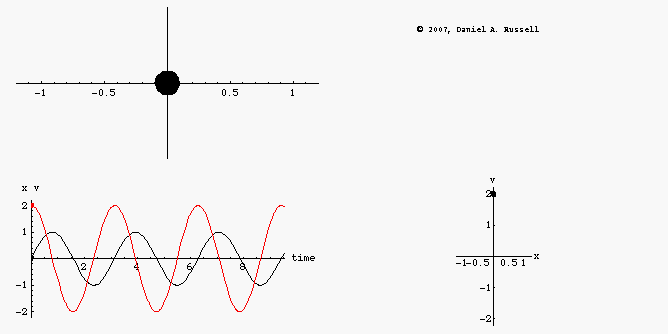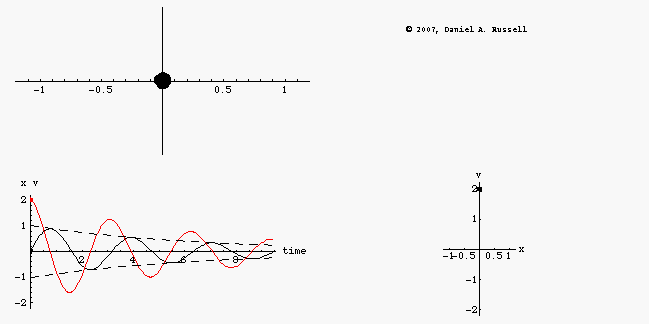Acoustics and Vibration Animations Daniel A. Russell, Ph.D. Graduate Program in Acoustics The Pennsylvania State University All text and images on this page are ©2004-2011 by Daniel A. Russell and may not used in other web pages or reports without permission.# Phase Space Diagrams for an Oscillator (undamped and damped)

When discussing oscillation, one often must consider both the displacement and velocity of the oscillator, especially when discussing potential energy (which depends on position) and kinetic energy (which depends on velocity). Both the displacement and velocity are functions of time and there is a 90o phase relationship between the two. A phase-space plot is a parametric graph of the velocity v(t) plotted as a function of the displacement x(t), with the changing variable being time. Phase-space plots are very useful for analyzing more complicated oscillations, especially oscillation that tends towards chaos. As such, they are often encountered in a study of classical (theoretical) mechanics.## Undamped Oscillator

The animations at left represents the motion of an undamped oscillator. The top left animation shows the object oscillating back and forth along the x-axis. The lower left animation is a plot superimposing the position x(t) as a function of time and the velocity v(t) as a function of time on the same graph. Dots have been provided to show how both the position and velocity alternate between positive and negative maximum values as the system oscillates. The combined plots also illustrate the difference in phase between position and velocity. When the position is maximum the velocity is passing through zero, and vice versa. However, the combined plot is not as easy to visualize without making it an animation. The phase-diagram plot, shown in the lower right, shows the phase relationship between velocity and position much more clearly. As the system oscillates, the phase diagram carves out a clockwise ellipse.## Damped Oscillator

When the oscillator has damping, the oscillator loses energy during each cycle, and both the position and velocity decrease in amplitude as time proceeds. Graphs of position versus time and velocity versus time display an amplitude envelope which decreases exponentially. The phase-space diagram for a damped oscillator also shows that the oscillator is losing energy. As the amplitudes of the velocity and position decrease, the phase diagram spirals inwards. In classical mechanics, this is described as an "attractor" and shows that the system is trapped in a potential well from which it cannot escape.

Back to the Acoustics and Vibration Animations page
` `